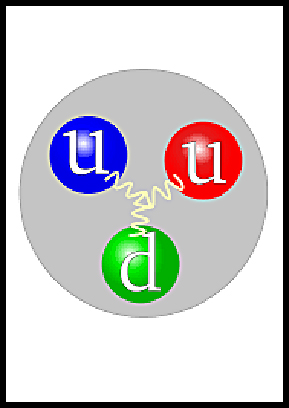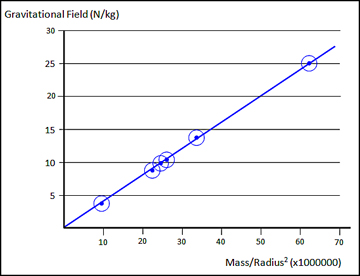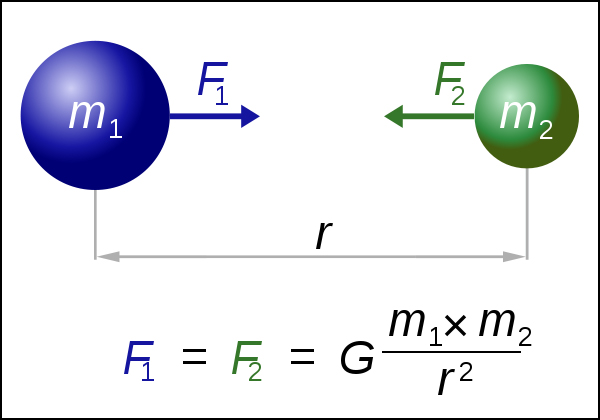# How Does Gravity Work

A possible solution to Gravity

What is gravity and how does it work? We all know its effects if we fall down or drop a brick on our toe. But science doesn’t know what makes gravity work. A theory that Albert Einstein postulated is that gravity isn’t a force but somehow distorts space and particles will move into this distortion. Say what? The simple explanation is that gravity sucks and attracts objects by the Law of Attraction. Which one makes more sense to you?Ouch, that hurts! Image from unsplash.com

## The Four Fundamental Forces

Scientists say there are 4 fundamental forces of nature, the weak nuclear force, the strong nuclear force, electromagnetism, and gravity.

I think that gravity is not a fundamental force. What did I say?

What I’m going to share with you is new information that deserves a Nobel Prize in physics, so call me maybe?

## We are part of this matter that has the intelligence to keep us alive. We would be fools if we think that the Big Bang came here by chance.

I am in awe of the creation that started the big bang and which continues to evolve around us. The mind is never matter and matter never minds (Erik Lovin 2019)

## The Movement of Energy During the Big Bang

At the start of the universe energy entered in the form of high-frequency electromagnetism.

The high-frequency radiation was massless and moved at the speed of light.

This light cooled down into a plasma of electrons and into quarks and gluons.

At CERN scientists have produced a plasma of quarks and gluons that has very little mass.

Apparently, these quantum particles have almost no mass when they are in the plasma state.

When temperatures cool quarks and gluons combine into quantum particles called hadrons.

Hadrons are made of 2 quarks and when they transition into 3 quarks they are called baryons which are protons and neutrons.

All matter is made of baryons containing protons and neutrons inside the nucleus of an atom. And all atoms have a mass and that mass has energy, E=mc^2 and that mass has a force called gravity.

## Gravity and The Big Bang Event

The energy that started the universe is often called the electro-weak era and it consists of electromagnetism of a very high frequency.

All of that initial energy was converted into electrons and then into the plasma of gluons and quarks.

When the plasma cooled the stable quarks attracted other quarks to make protons which are ionized hydrogen atoms.

Hydrogen atoms were created when these positively charged particles attracted negatively charged electrons and the universe has started.

During the creation of protons, quantum gravity emerged which is called the 4th-fundamental force.

Quantum gravity is born by the interaction of quarks and gluons and gravity is formed when quarks attract more quarks.

Eventually, when enough hydrogen atoms are attracted into one big cloud a nuclear reaction ignites it into a star and light is born. Let there be light.

The main takeaway is that gravity is an emergent force from the quantum reactions of quarks attracting more quarks.

## Quantum Gravity in Quarks

Quarks are held together by the strong nuclear force which is mediated and provided by gluons.

So quarks use a force called a color charge that makes them engage in the strong nuclear force.

This color force is controlled by a quark/gluon interaction called quantum chromodynamics QCD.

The color force is the same force that’s called the strong nuclear force. But this force is always moving inside quarks to maintain a neutral colour charge.

The force also acts as an attractive force to any matter that has quarks, so quarks are attracted to other quarks.

Gluons and quarks don’t exist on their own, and they are only found inside matter.

Gluons hold 3 quarks together to make a proton or a neutron, and they also hold the nucleus together inside the atom. Eureka!

Gluons hold stuff together! Gravity holds stuff together. This isn’t rocket science, it’s the logic of quantum physics.

There is no doubt that gravity is a property of mass! The gluon particle holds quarks together and quarks attract more quarks obviously using quantum gravity.

The mass of atoms and molecules is about 99% from the attractive binding force of the gluon energy. According to Einstein energy can be expressed as mass, E=mc^2.

## What is Gravity Made from

Gluons are the strong nuclear force that holds quarks together to make protons and neutrons. When protons attract electrons stable atoms are created.

The Gluon force is kind of like a spring attached between two quarks. During molecular vibration this spring keeps a constant force binding the quarks together.

There is also a color force that mediates the strong force associated with this interaction.

The force of the spring analogy explains how it seems to work. If you try to separate quarks from the gluons the spring becomes stronger and prevents them from pulling apart.

Adding enough energy to force them apart changes the extra energy into new quark pairs. So you created more matter from energy but it’s impossible to get free quarks.

Gluons and quarks are never free particles (except in the quark-gluon plasma during the big bang).

Furthermore, the colour force (the strong nuclear force) has 3 ranges of strength.The proton has two up quarks and one down quark with a neutral colour force (rgb) held together by 3 gluons giving it a positive charge.

The quark to quark bond is the first range for gluons at the quantum distance of quarks.

At a slightly longer distance, gluons hold the nucleus together that contains various protons and neutrons.

Otherwise, the protons would repel each other and fly out of the nucleus. Then the 3rd range is quantum gravity.

### Quantum Gravity from Quarks/Gluons

Gluons have a 3rd range that extends beyond the nucleus. Beyond the nucleus range, there is an attraction to other atoms that have quarks.

This attraction to nearby quarks explains why the force of gravity depends on the mass of the object.

The quantum force of attraction is too small to measure on the level of single atoms but gravity is an additive force.

When the number of atoms adds up to the size of large gaseous clouds the combined force of gravity starts a nuclear fusion reaction.

Isn’t it amazing that gluons create and attract matter using the strong nuclear force? Then gravity (from gluons) attracts enough matter to create a nuclear fusion reaction.

This fusion reaction creates many more types of matter during a star’s supernova reaction.

## Gravity works by using Gluons

Gluons create quantum gravity by interacting with quarks. Therefore, matter must have quarks in order to have a force of gravity and all Baryonic matter have quarks.

Thus, all other fundamental particles lack gravity. Electrons, neutrinos, and photons can have mass but not gravity.

That means they are attracted to gravity but are not attracted to each other since they don’t have a field of gravity; namely quarks/gluons.

## The Theory of Everything (TOE)

The information in this article explains the way gravity works in the quantum world. The additive force of Gravity explains why it also works on the large scale of the Universe.

Adding the quantum gravity theory to Newtonian gravity gets us close to the Theory of Everything. A Nobel Prize?

## The Planets and the Earth’s Gravity

The graph below shows that the gravitational force of each planet can be calculated from the linear curve if you know the mass and radius of the planet.Image thanks to schoolphysics.co.uk

The force of gravity on a planet depends on the mass (M) but also on the size (r) of the massThe radius of an object is needed because the mass in the equations is concentrated in the center of the mass.

One idea that I have is to calculate the force on one mole of a pure element such as iron, gold or silver (9.81 Newtons) and determine the number of quarks in that Avogadro number.

Then calculate the g force on each quark-gluon particle to see how quantum gravity adds up to equal gravitational force g.

Does anyone know how to do this and test it on many atoms for accuracy? If you feel lucky, call me maybe? We need to talk.  elovin@studioeurope.ca  https://lovinthings.com